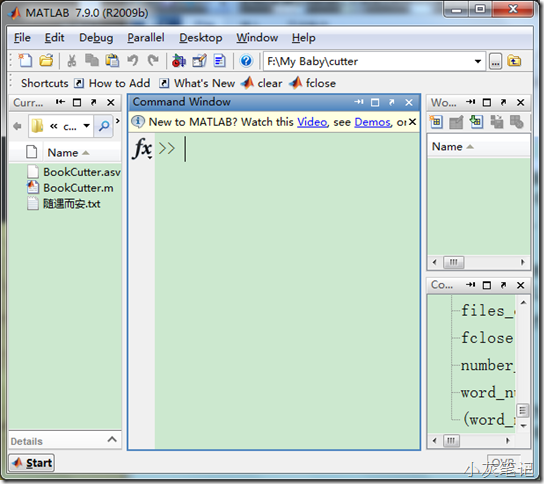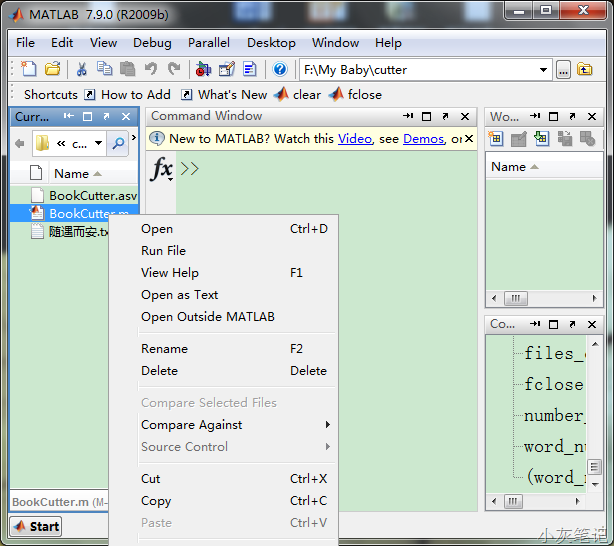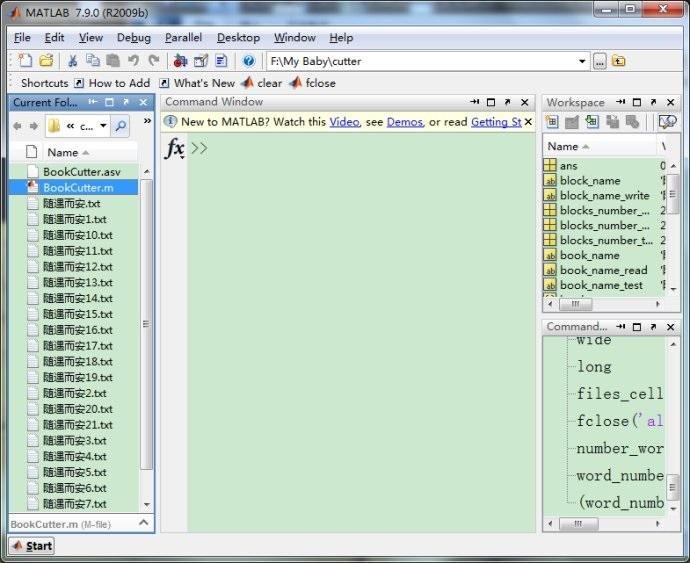您可以捐助，支持我们的公益事业。 1元 10元 50元 认证码：必填求知 文章 文库 Lib 视频 iPerson 课程 认证 咨询 工具 讲座 Modeler Code要资料订阅捐助

 评价： 好 中 差

1.把脚本cutter.m跟要切割的电子书放到matlab的工作路径之下；

2.运行脚本，OK。```clear; clc; files_cell = ls; size_files = size(files_cell); long = size_files(2); wide = size_files(1); word_number_block = 5000; file_name = ''; text_block = ''; k = 0; for i = 1:wide for j = 1:long if long ~= j file_name = strcat(file_name,files_cell(i,j)); else book_name_test = file_name; file_name = ''; txt_match = regexp(book_name_test,'.txt','match'); if ~isempty(txt_match) book_name_txt = regexp(book_name_test,'.txt','split'); book_name = char(book_name_txt{1,1}); break; end end end end book_name_read = strcat(book_name,'.txt'); text = fileread(book_name_read); number_word = length(text); blocks_number_cal = number_word/word_number_block; blocks_number_test = fix(number_word/word_number_block); if blocks_number_test == blocks_number_cal blocks_number_real = blocks_number_test; elseif blocks_number_test < blocks_number_cal blocks_number_real = blocks_number_test + 1; end for i = 1:blocks_number_real block_name = strcat(book_name,int2str(i)); block_name_write = strcat(block_name,'.txt'); fid = fopen(block_name_write,'w'); for j = (word_number_block*(i-1)+1):(word_number_block*(i-1)+5001) k = k+1; if k <= number_word fprintf(fid,'%s',text(k)); else break; end if word_number_block*(i-1)+5001 == j fclose(fid); end end end fclose('all'); ```

 评价: 好 中 差订阅捐助
 相关文章 UML概览 UML图解:用例图（Use case diagram ） UML图解:活动图（activity diagram ） UML图解:类图（class diagram ）
 相关文档 UML统一建模语言参考手册 网上商城UML图制 UML建模示例：JPetStor UML序列图编写规范
 相关课程 UML与面向对象分析设计 UML + 嵌入式系统分析设计 业务建模与业务分析 基于UML和EA进行系统分析设计讲座 小米业务部门的敏捷转型 主讲：杜伟忠 时间：2020-6-13实录 精益产品生命周期管理 主讲：杨云

 如何向妻子解释OOD OOAD与UML笔记 UML类图与类的关系详解 UML统一建模语言初学 总结一下领域模型的验证 基于 UML 的业务建模

 面向对象的分析设计 基于UML的面向对象分析设计 UML + 嵌入式系统分析设计 关系数据库面向OOAD设计 业务建模与业务架构 使用用例进行需求管理

 某航空IT部门 业务分析与业务建模 联想 业务需求分析与建模 北京航管科技 EA工具与架构设计 使用EA和UML进行嵌入式系统分析 全球最大的茶业集团 UML系统分析 华为 基于EA的嵌入式系统建模 水资源服务商 基于EA进行UML建模